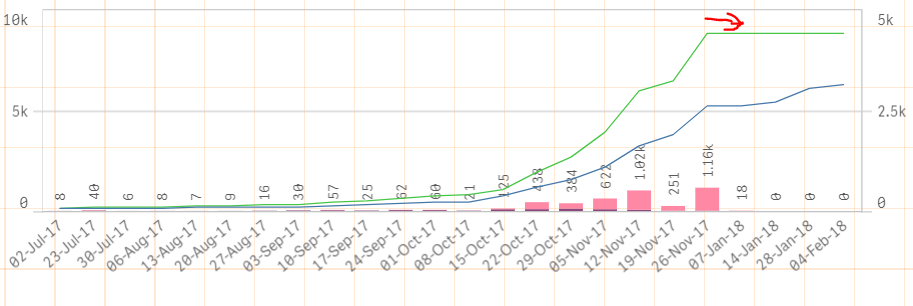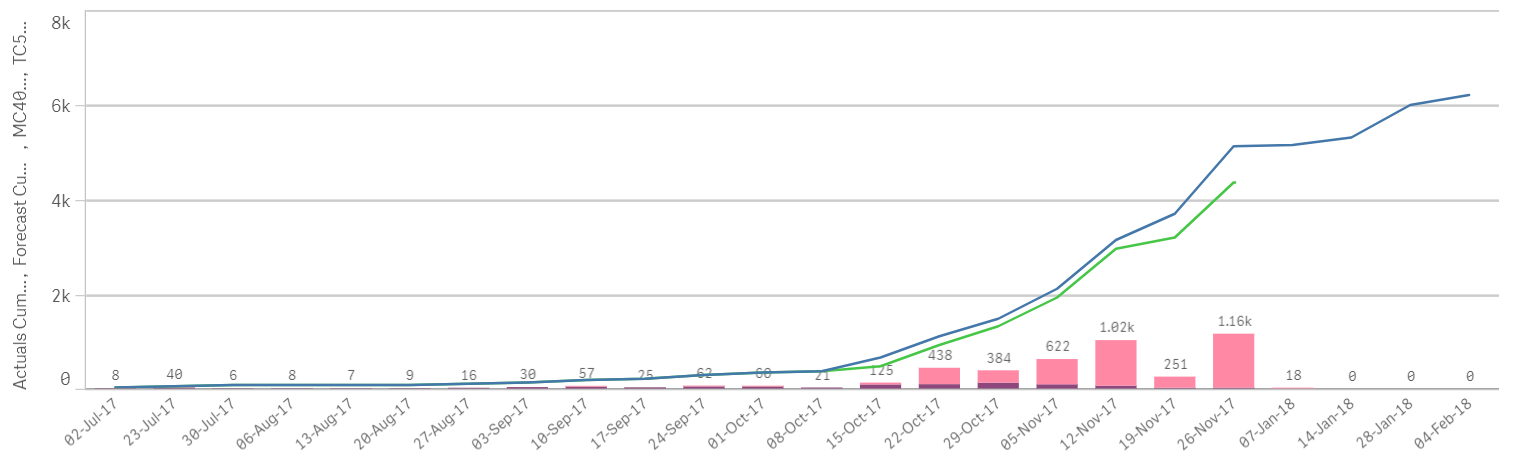Announcements
Applications are open for the 2024 Qlik Luminary Program. Apply by December 15 here.
cancel
Showing results for
Did you mean:Partner - Creator

## Stopping rangesum showing after a given date

Hi

I am using rangesum function to plot Forecast vs Actuals along the same date line. When i run the Actual sum range, it works by restricting the date based on the set analysis (see formula below). However I want to also show forecast numbers for the next couple of weeks after the actuals have ended (ie "today"). When I do this it shows the actuals as a flat line across those dates (see image). Is there a way to stop the actuals showing for dates "after today"

Actual: rangesum(above( sum({<[Date]={"<\$(Today())"}>}[Actual_deployment]),0,rowno()))Rakesh

1 Solution

Accepted SolutionsMVP

try; if (sum([Actual_deployment])=0,null(),

rangesum(above( sum({<[Date]={"<\$(Today())"}>}[Actual_deployment]),0,rowno())))

this will work

7 RepliesMaster II

Hi,

You can try like below,

Rangesum(above(sum({\$<DATE = {'\$(=Date(Today() ))'}>}[Actual_deployment]),0,rowno()))

or

Rangesum(above(sum({\$<Date = {"=\$(=Date(Today(), 'MM/DD/YYYY'))"}>}[Actual_deployment]),0,rowno()))

Thanks,

DevaPartner - Creator
Author

Hi Deva, thank you for your reply. This sort of works... as the only measure in the graph it will reduce the dates dimension to less than today (btw had to a '<' into your formula)... however when you add the forecast measure it adds the future dates in (as expected) and the line flattens across. Trying to remove the flattening line on future dates.

Thanks

RakeshMaster II

Hi,

after adding the less than Today. is it working?

Rangesum(above(sum({\$<Date = {"<=\$(=Date(Today(), 'MM/DD/YYYY'))"}>}[Actual_deployment]),0,rowno()))Partner - Creator
Author

the set analysis is working, but its not displaying how I want it to.

will demonstrate with images

How it is currently showingHow I would like it to showMaster II

Hi,

can you send me forecast  expression? also would be able to share your mock data with app. thanks

may be u can try this

Less than today set condition apply only your  actual measure field

forecast just remove that set analysis part like below,

Rangesum(above(sum(Forecast_Amt]),0,rowno()))

Thanks,

DevaMVP

try; if (sum([Actual_deployment])=0,null(),

rangesum(above( sum({<[Date]={"<\$(Today())"}>}[Actual_deployment]),0,rowno())))

this will workPartner - Creator
Author

Awesome thanks Omar and Deva for your help.

The combination has resolved it - but namely the logic of if(sum)Tags
Community Browser